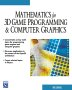# Maths BooksThe Princeton Companion to Mathematics - This is a big book that attempts to give a wide overview of the whole of mathematics, inevitably there are many things missing, but it gives a good insight into the history, concepts, branches, theorems and wider perspective of mathematics. It is well written and, if you are interested in maths, this is the type of book where you can open a page at random and find something interesting to read. To some extent it can be used as a reference book, although it doesn't have tables of formula for trig functions and so on, but where it is most useful is when you want to read about various topics to find out which topics are interesting and relevant to you.Handbook of Mathematics - This is a really useful reference book where you can find formulas, definitions and descriptions of a wide range of mathematics. The book is very nicely bound with a flexible plastic cover.Visual Complex Analysis - If you already know the basics of complex numbers but want to get an in depth understanding using an geometric and intuitive approach then this is a very good book. The book explains how to represent complex transformations such as the Möbius transformations. It also shows how complex functions can be differentiated and integrated.Clifford Algebra to Geometric Calculus: A Unified Language for Mathematics and Physics (Fundamental Theories of Physics). This book is intended for mathematicians and physicists rather than programmers, it is very theoretical. It covers the algebra and calculus of multivectors of any dimension and is not specific to 3D modelling.Clifford Algebras and Spinors (London Mathematical Society Lecture Note S.)   Pertti Lounesto. This is very complex subject matter, however there is a lot of explanations and it is not all proofs like some mathematical textbooks. The book has a lot of information about the relationship between Clifford Algebras and Hypercomplex Algebras.Geometric Algebra for Physicists - This is intended for physicists so it soon gets onto relativity, spacetime, electrodynamcs, quantum theory, etc. However the introduction to Geometric Algebra and classical mechanics is useful.Geometric Algebra for Computer Science: An Object-oriented Approach to Geometry. This book stresses the Geometry in Geometric Algebra, although it is still very mathematically orientated. Programmers using this book will need to have a lot of mathematical knowledge. Its good to have a Geometric Algebra book aimed at computer scientists rather than physicists. There is more information about this book here.

metadata block
see also:

PhysicsWWW https://www.euclideanspace.com
Correspondence about this page

Book Shop - Further reading.

Where I can, I have put links to Amazon for books that are relevant to the subject, click on the appropriate country flag to get more details of the book or to buy it from them.Mathematics for 3D game Programming - Includes introduction to Vectors, Matrices, Transforms and Trigonometry. (But no euler angles or quaternions). Also includes ray tracing and some linear & rotational physics also collision detection (but not collision response).

other books

This site may have errors. Don't use for critical systems.

Copyright (c) 1998-2021 Martin John Baker - All rights reserved - privacy policy.